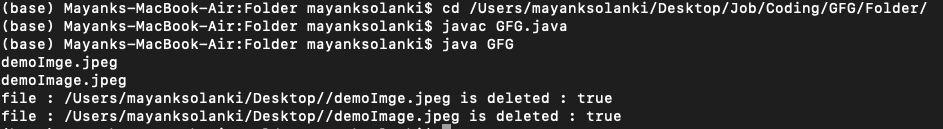Open in App
Not now

# Java Program to Find/Delete Files Based on Extensions

• Last Updated : 10 Aug, 2021

We can use FilenameFilter in Java to override the accept(File dir, String name) method to execute the file filtering operation. Filename interface has a method accept(). It checks whether a given file should be included in a list of files. We can check each file in the passed directory by implementing this method. If the file has the required extension, then it is included otherwise discarded.

Syntax:

`accept(File dir, String name)`

Now the next things that come into play is how to include the files in a list with the given extension, for that there is a File.list() method that takes the FilenameFilter instance. the list Returns an array of strings naming the files and directories in the directory denoted by this abstract pathname that satisfies the specified filter. So let us discuss this function in detail to get a better understanding while dealing with the files.

The list() method is a part of File class. The function returns an array of the string denoting the files in a given abstract pathname if the pathname is a directory else returns null. The function is an overloaded function. One of the function does not have any parameter and the other function takes FilenameFilter object as a parameter as described below

Function Signature:

```public String[] list()
public String[] list(FilenameFilter f)```

Function Syntax:

```file.list()
file.list(filter)```

Parameters: The function is an overloaded function. One of the function does not have any parameter and the other function takes FilenameFilter object as a parameter

Return value: The function returns a string array, or a null value if the file object is a file.

Exception: This method throws Security Exception if the function is not allowed to write access to the file.

Procedure:

We will follow two steps to delete files based on extensions:

1. Implement the FileNameFilter interface to list files with the given extension.
2. Iterate through those files to delete them using the delete() method.

Example:

## Java

 `// Java Program to find/delete files based on extensions `   `// Importing input output classes` `import` `java.io.*;`   `// Class 1` `// Main class` `// To check for a file` `class` `GFG {`   `    ``// Member variables of this class`   `    ``// File directory` `    ``private` `static` `final` `String FILE_DIRECTORY` `        ``= ``"/Users/mayanksolanki/Desktop/"``;` `    ``// File extension` `    ``private` `static` `final` `String FILE_EXTENSION = ``".jpeg"``;`   `    ``// Method of this class` `    ``public` `void` `deleteFile(String folder, String extension)` `    ``{`   `        ``// Creating filter with given extension by` `        ``// creating an object of FileExtFilter` `        ``FileExtFilter filter = ``new` `FileExtFilter(extension);`   `        ``// Now, creating an object of FIle  class` `        ``File direction = ``new` `File(folder);`   `        ``// Cresting an array if strings to` `        ``// list out all the file name` `        ``// using the list() with .txt extension` `        ``String[] list = direction.list(filter);`   `        ``// Iterating over the array of strings` `        ``// using basic length() method` `        ``for` `(``int` `i = ``0``; i < list.length; i++) {` `            ``// printing the elements` `            ``System.out.println(list[i]);` `        ``}`   `        ``// Base condition check when array of strinfg is` `        ``// empty Then simply return` `        ``if` `(list.length == ``0``)` `            ``return``;`   `        ``File fileDelete;`   `        ``// Now looking for the file  in the` `        ``for` `(String file : list) {`   `            ``String temp = ``new` `StringBuffer(FILE_DIRECTORY)` `                              ``.append(File.separator)` `                              ``.append(file)` `                              ``.toString();`   `            ``// Storing the file` `            ``fileDelete = ``new` `File(temp);`   `            ``// Checking whether the file is deleted` `            ``boolean` `isdeleted = fileDelete.delete();`   `            ``// Print true if file is deleted` `            ``System.out.println(``"file : "` `+ temp` `                               ``+ ``" is deleted : "` `                               ``+ isdeleted);` `        ``}` `    ``}`   `    ``// Method 2` `    ``// Main driver method` `    ``public` `static` `void` `main(String args[])` `    ``{`   `        ``// Calling the deleteFile() method over the file` `        ``// FileCheker() method to check existence for the` `        ``// file`   `        ``// Delete the file with FILE_EXTENSION from` `        ``// FILE_DIRECTORY using the deleteFile() method s` `        ``// created above` `        ``new` `GFG().deleteFile(FILE_DIRECTORY, FILE_EXTENSION);` `    ``}` `}`   `// Class 2` `// Helper class` `// Which in itself is implementing FilenameFilter Interface` `class` `FileExtFilter ``implements` `FilenameFilter {`   `    ``// Extension` `    ``private` `String extension;`   `    ``// Comparator` `    ``public` `FileExtFilter(String extension)` `    ``{`   `        ``// This keyword refers to current object itself` `        ``this``.extension = extension;` `    ``}`   `    ``public` `boolean` `accept(File directory, String name)` `    ``{`   `        ``// Returning the file name along with the file` `        ``// extension type` `        ``return` `(name.endsWith(extension));` `    ``}` `}`

Output:My Personal Notes arrow_drop_up
Related Articles